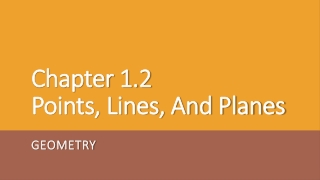Download PresentationChapter 1.2 Points, Lines, And Planes

# Chapter 1.2 Points, Lines, And Planes

Download Presentation## Chapter 1.2 Points, Lines, And Planes

- - - - - - - - - - - - - - - - - - - - - - - - - - - E N D - - - - - - - - - - - - - - - - - - - - - - - - - - -
##### Presentation Transcript

1. Point: • A Location in Space • Has No Size • Name a point with a CAPITAL LETTER and represent it with a dot • Line: • Straight Path of Points • No Thickness • Extends Infinitely in Opposite Directions t A B

2. A C • Collinear: • Three or more points on a line. • Noncollinear: • Points do not form a line. B ● ● B ● ● ● ● A C

3. Ex.1) Identify Collinear Points • Are the following points collinear? • A, E, C • G, F, B • D, E, A • Name line m in three other ways • Name line l in three other ways

4. PLANE R . S . T . X • Flat Surface/No Thickness • Infinite Number of Points and Lines • Extends Without End • Any 3 Noncollinear Points ALWAYS Make a Plane • Name a Plane • A Capital Letter or • At Least 3 of its Noncollinear Points Plane RST or Plane X

5. Ex.2) Name the Plane The Bottom The Top The Front The Back The Left Side The Right Side A B D C E F H G

6. COPLANAR R .S . T. X Lines or points in the same plane.

7. t A B P ● m n Line t is the only line that passes through points A and B. Postulate 1-1 Two lines intersect in exactly one point. Lines m and n intersect at point P. Postulate 1- 2 Through any 2 points there is exactly one line.

8. A line and a plane intersect, in exactly one point.

9. Postulate 1-3 ●A ●B ●C D t Plane ABC and Plane D intersect at line t. Two planes intersect, in exactly one line.

10. M P L Picture this cube as having Planes M, L and P. They intersect in 3 separate lines.

11. Ex.3) Name the intersection of Planes ABC & BFG Name two planes that intersect the given line. A B D C E F H G

12. Can you name sets of noncollinear points? A B D C E F Postulate 1-4 Through any 3 noncollinear points there is exactly one plane. H G

13. A B D C E F Find Planes: ABC HEF BCG ADG H G

14. Ex.4) • Shade the plane containing points A, E, F • Name another point in Plane EHG • Is point F coplanar with plane DCG? A B D C E F H G